# G-filtered Polycules

The strongest thing i can say about G-filtered Polycules, is that "someday, grade school kids will be rational-factoring cubic polynomials without even knowing it". So you can only imagine what they will be capable of when they enter High School, College, and Beyond, and NOT just in Mathematics !!! A design for the "game" they play after recess might look like the image below, "CUBICS CUBE" sounds catchy for a title. Find $m$ and the factors of the cubic $x^3-3x^2-11x+21$ magically appear, the BIG CIRCLE equation reveals the Rational factor, and the LITTLE CIRCLE equation reveals the quadratic factor.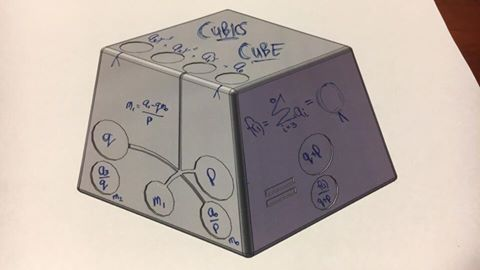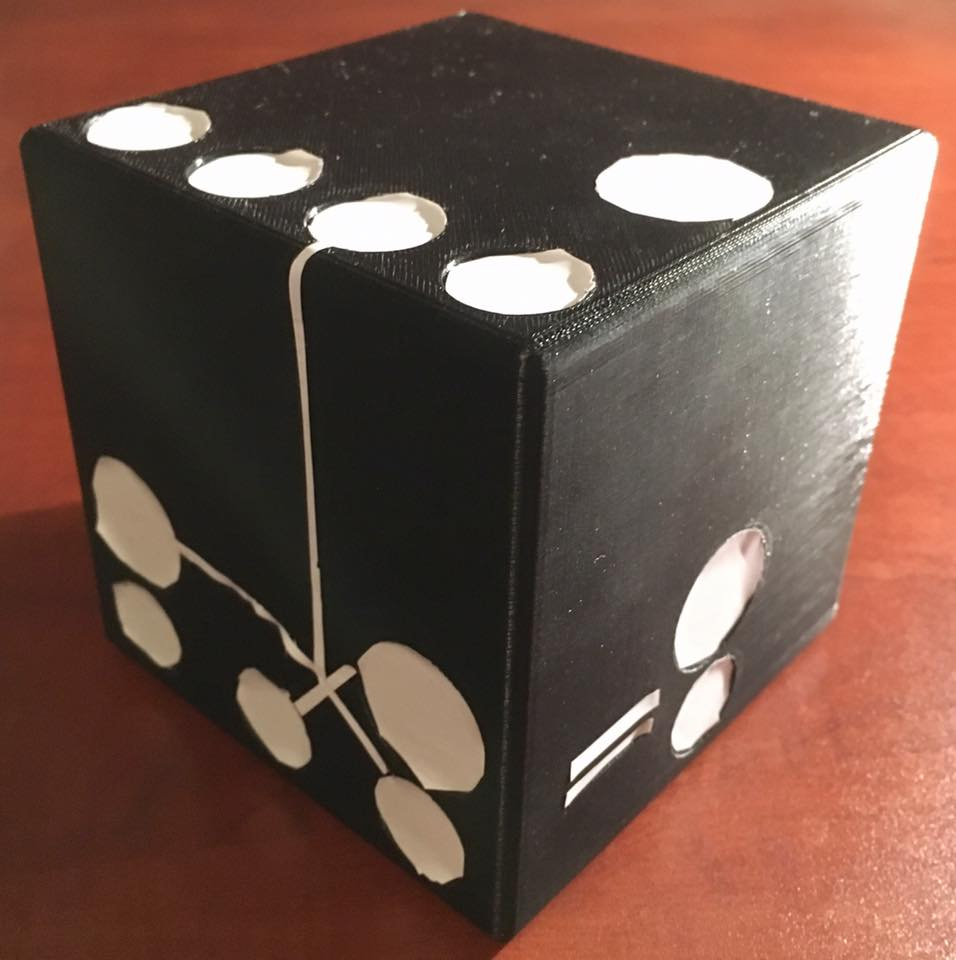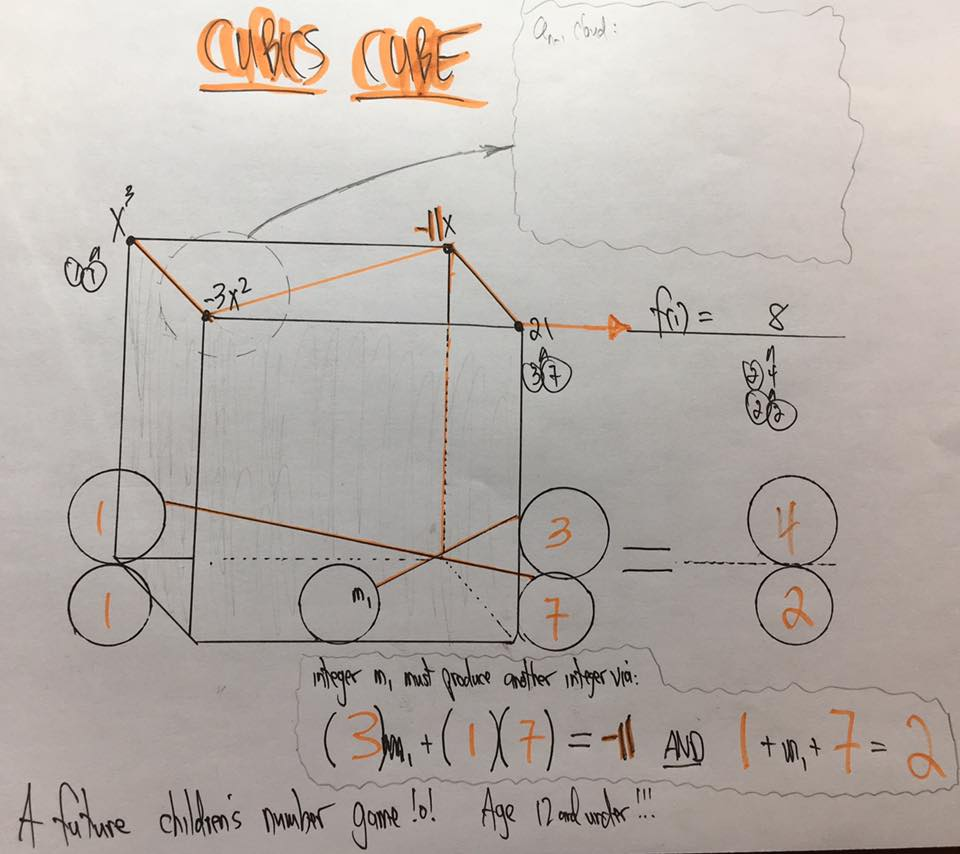The pic below shows the formal Mathematics in factoring $x^3-3x^2-11x+21$ via Unit Conjugate G-filtration. Note: DROS is Descartes' Rule Of Signs, which when used with Conjugate G-filtration makes a powerful factoring Tool for ANY polynomial !!!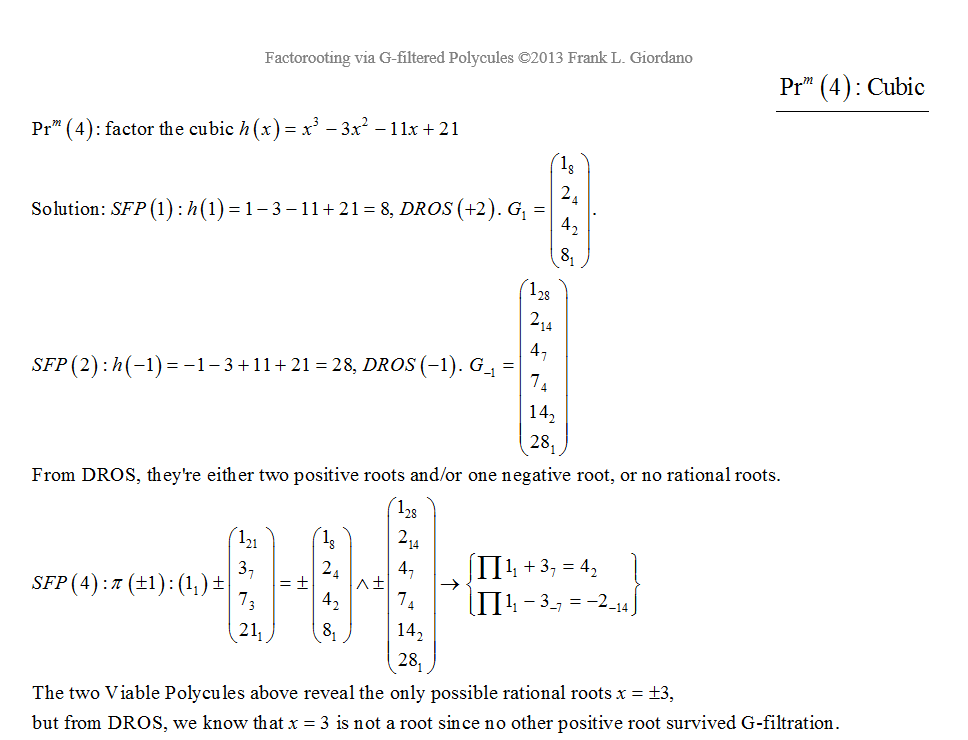Junior High School grads can now factor an integer-coefficient polynomial of ANY degree. The pic below shows the work required in factoring the cubic $2x^3-7x^2-16x+35$. How would you find all the rational factors ??? This facebook video explains the Game of G-filtered Polycules for Cubics; leave a comment:

https://www.facebook.com/TruSpot/videos/vb.1021243805/10208395010805791/?type=2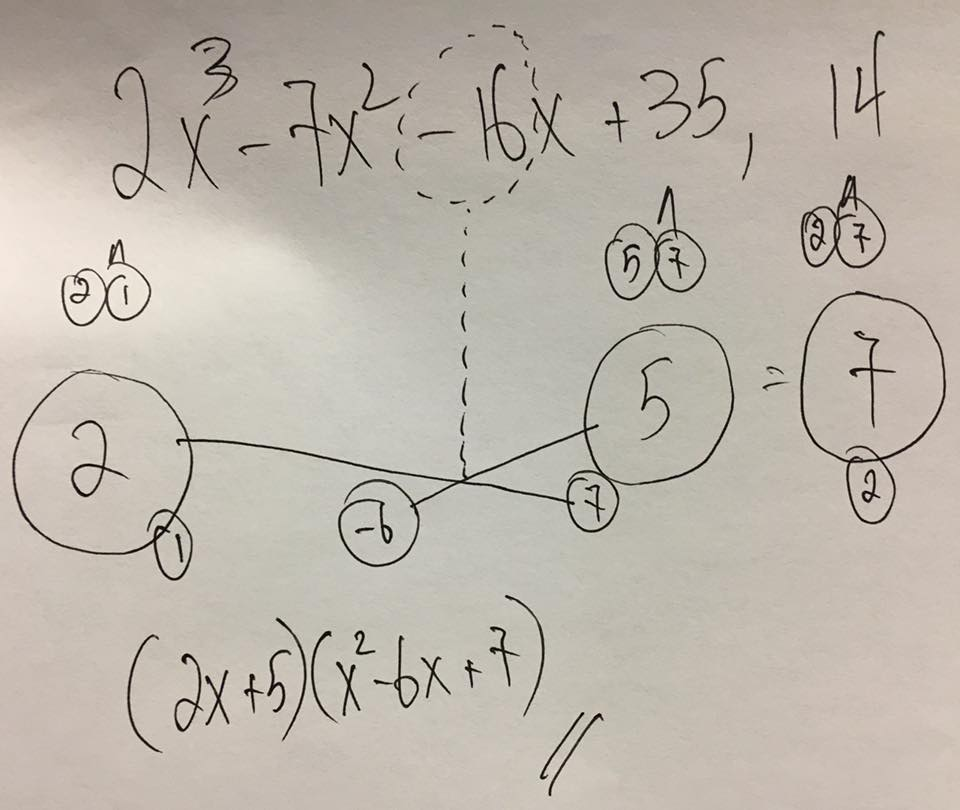And here is the Introduction to my Book(1), and below that, the Quadratic $x^2-7x+12$ factored via Unit G-filtration.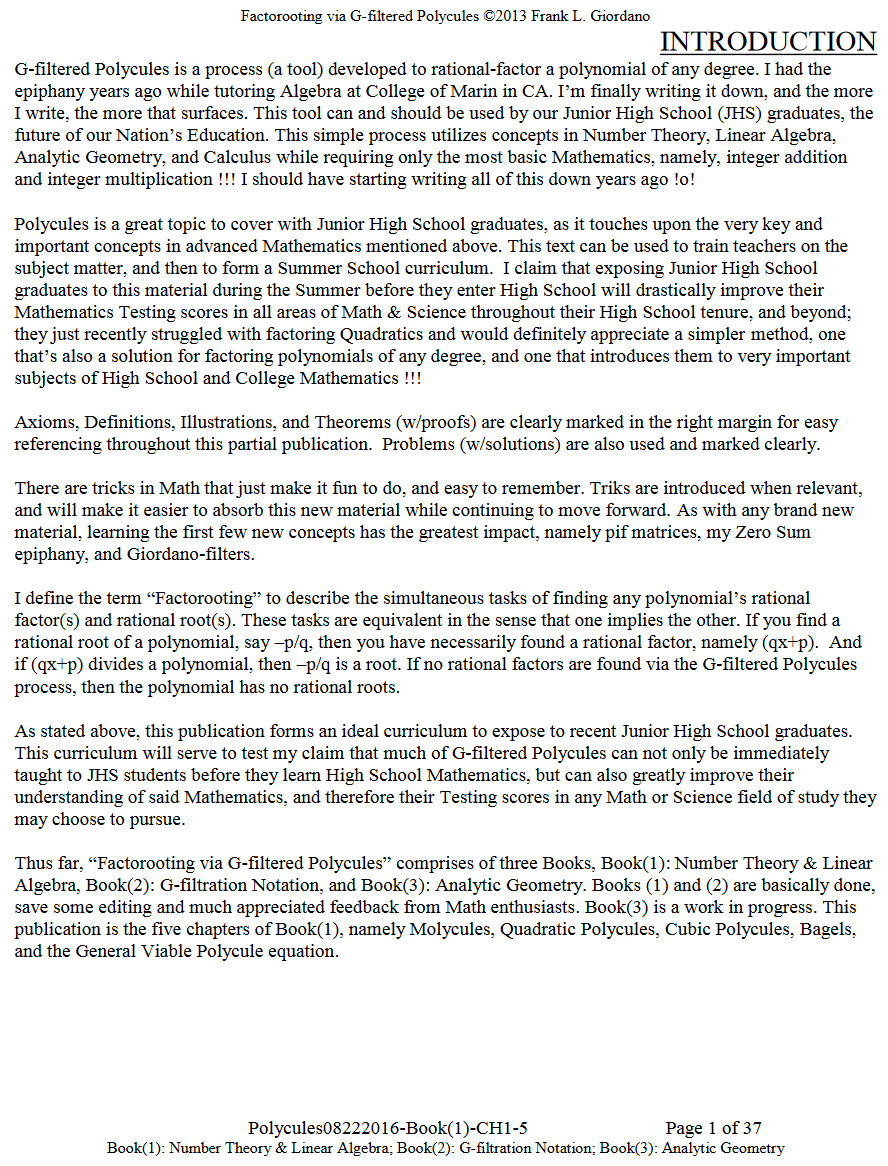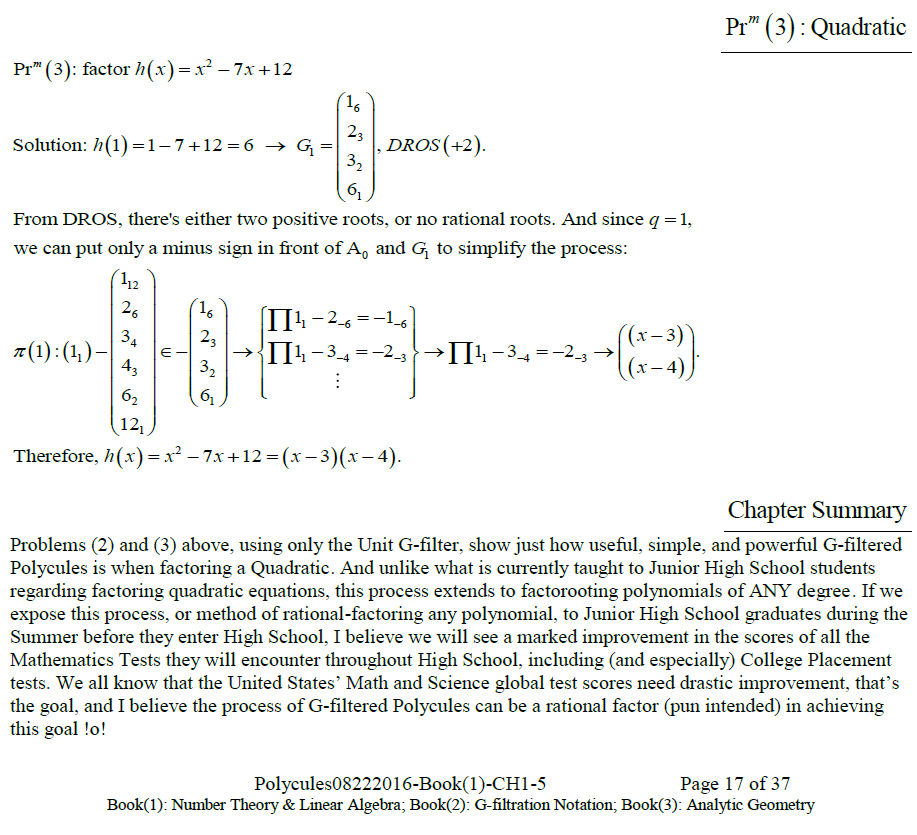And finally, this is the "G-filtration Cumulative Summary" from Book(1):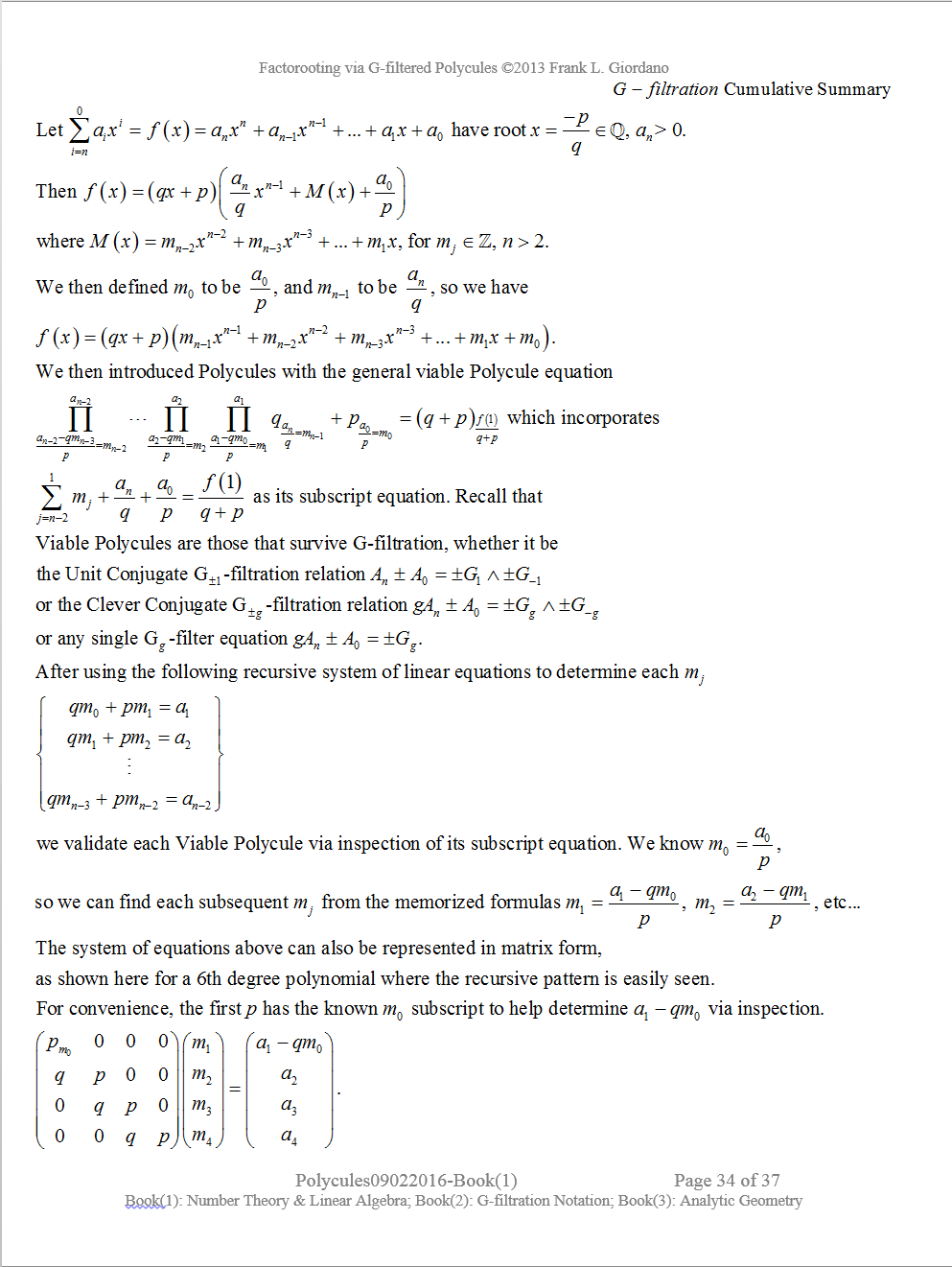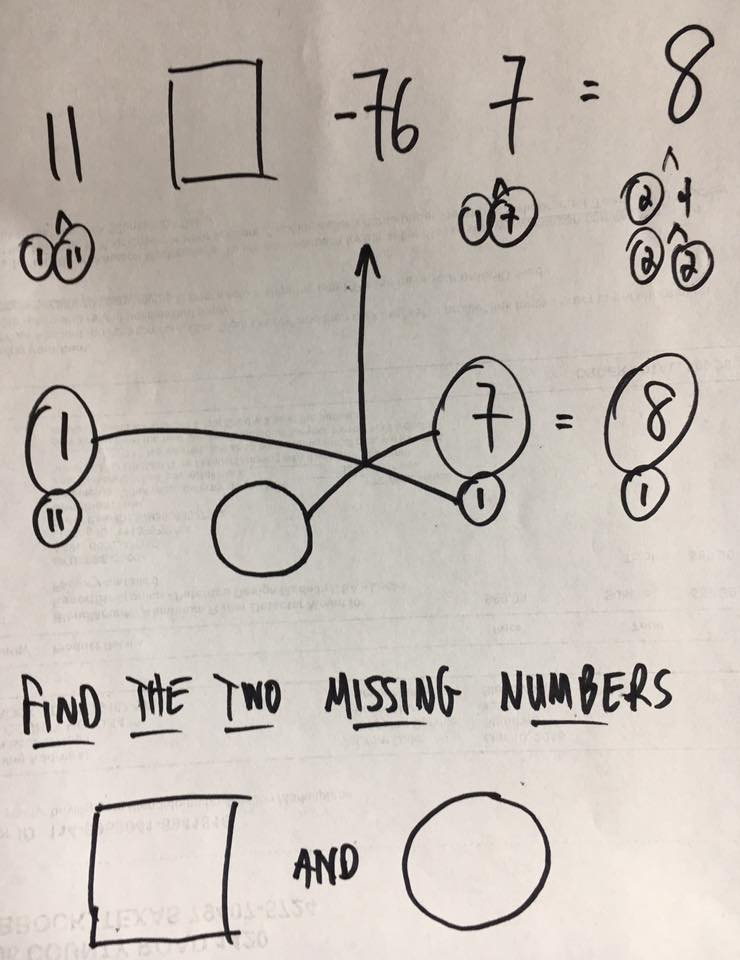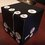Note by Frank Giordano
3 years, 5 months ago

This discussion board is a place to discuss our Daily Challenges and the math and science related to those challenges. Explanations are more than just a solution — they should explain the steps and thinking strategies that you used to obtain the solution. Comments should further the discussion of math and science.

When posting on Brilliant:

• Use the emojis to react to an explanation, whether you're congratulating a job well done , or just really confused .
• Ask specific questions about the challenge or the steps in somebody's explanation. Well-posed questions can add a lot to the discussion, but posting "I don't understand!" doesn't help anyone.
• Try to contribute something new to the discussion, whether it is an extension, generalization or other idea related to the challenge.

MarkdownAppears as
*italics* or _italics_ italics
**bold** or __bold__ bold
- bulleted- list
• bulleted
• list
1. numbered2. list
1. numbered
2. list
Note: you must add a full line of space before and after lists for them to show up correctly
paragraph 1paragraph 2

paragraph 1

paragraph 2

[example link](https://brilliant.org)example link
> This is a quote
This is a quote
    # I indented these lines
# 4 spaces, and now they show
# up as a code block.

print "hello world"
# I indented these lines
# 4 spaces, and now they show
# up as a code block.

print "hello world"
MathAppears as
Remember to wrap math in $$ ... $$ or $ ... $ to ensure proper formatting.
2 \times 3 $2 \times 3$
2^{34} $2^{34}$
a_{i-1} $a_{i-1}$
\frac{2}{3} $\frac{2}{3}$
\sqrt{2} $\sqrt{2}$
\sum_{i=1}^3 $\sum_{i=1}^3$
\sin \theta $\sin \theta$
\boxed{123} $\boxed{123}$

Sort by:

- 3 years, 3 months ago

- 3 years, 5 months ago

Where are the books (1) and (2) available?

- 3 years, 5 months ago

- 3 years, 5 months ago

Can you provide a brief step-by-step explanation to this method? This is hard to understand. But I find this method much efficient than rational root theorem.

- 3 years, 5 months ago